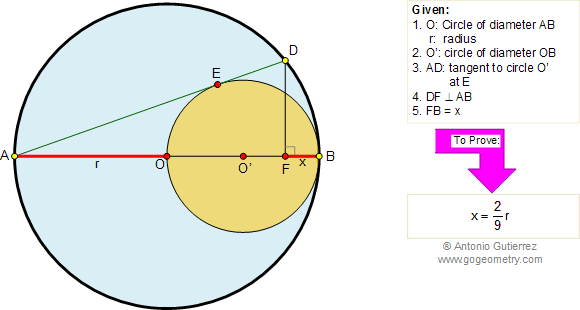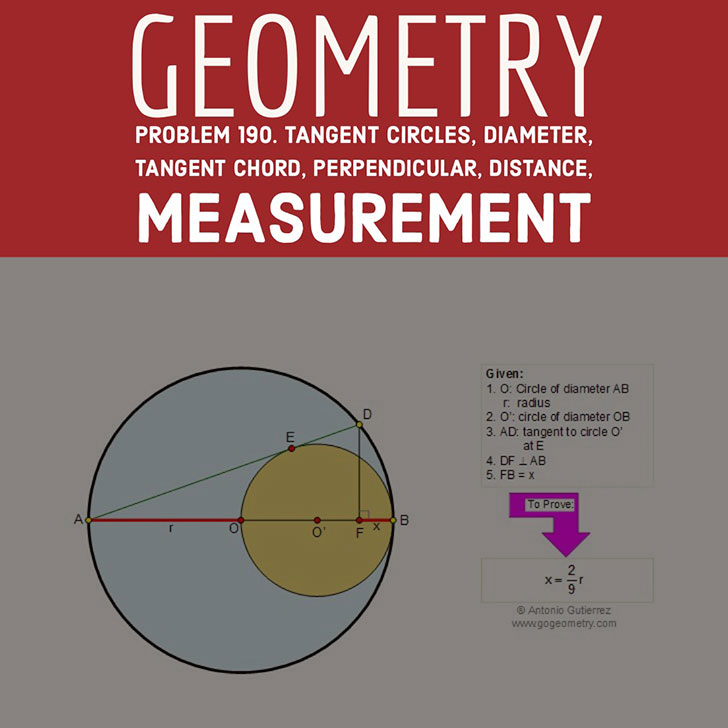Problem 190. Tangent circles, Tangent chord, Perpendicular, Diameter, Distance

 In the figure below, AB is the diameter of circle of center O and radius r. OB is the diameter of circle of center O'. The chord AD is tangent to circle O' at E. If DF is perpendicular to AB and BF = x, prove that. View or post a solution.## Poster and Typography of Problem 190Home | Geometry | Search | Problems | 181-190 | Semicircle | Email